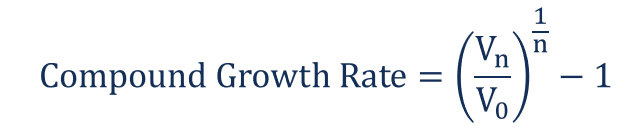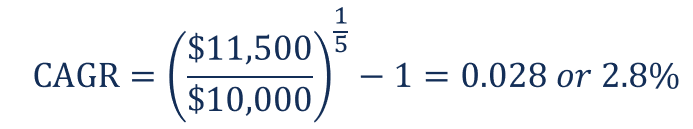Compound Growth Rate

A measure used to determine the growth rate over multiple time periods

What is the Compound Growth Rate?

The compound growth rate is a measure used specifically in business and investing contexts that determines the growth rate over multiple time periods. It is a measure of the constant growth of a data series. The biggest advantage of the compound growth rate is that the metric takes into consideration the compounding effect. Thus, it is especially significant in the assessment of returns from investments.Unlike average growth rates that are prone to volatility levels, compound growth rates are not affected by volatility. Therefore, it is more relevant in the comparison of different data series.

Understanding the Compound Annual Growth Rate (CAGR)

The compound annual growth rate (CAGR) is one of the most frequently used metrics in financial analysis and financial modeling. In financial models, the CAGR is calculated for important operational metrics such as EBITDA, and also for capital expenditures (capex) and revenue. Also, the CAGR can be used for the forecasting of future growth rates.

However, one should be careful in using the compound growth rate in financial analysis. The metric smooths the historical data, omits the effect of volatility, and implies the steady growth of the data series. Due to those reasons, the compound growth rate should best be used together with some other metrics, and the context of the given situation must always be considered.

How to Calculate the Compound Growth Rate?

The formula for calculating the compound growth rate is:Where:

• V– the ending value
• V– the beginning value
• n – the number of periods

Example of Compound Growth Rate

Five years ago, Sam invested \$10,000 in the stocks of ABC Corp. Below, you can see the total value of his investment at the end of each year:

• Year 1: \$10,500
• Year 2: \$8,500
• Year 3: \$9,750
• Year 4: \$10,700
• Year 5: 11,500

Sam wants to determine the steady growth rate of his investment. In such a case, the steady growth rate is equal to the compound annual growth rate (CAGR). The CAGR of his investment is calculated in the following way:Over the five-year period, Sam’s investment grew by 2.8%. Please note that we used \$10,000 as the beginning value because it was the amount of the initial investment.

Thank you for reading CFI’s explanation of the compound growth rate. CFI offers the Financial Modeling & Valuation Analyst (FMVA)™ certification program for those looking to take their careers to the next level. To keep learning and advancing your career, the following resources will be helpful:

• CAGR Calculator
• Capital Expenditures
• VIX
• Year over Year (YOY)

Financial Analyst Training

Get world-class financial training with CFI’s online certified financial analyst training program!

Gain the confidence you need to move up the ladder in a high powered corporate finance career path.

Learn financial modeling and valuation in Excel the easy way, with step-by-step training.